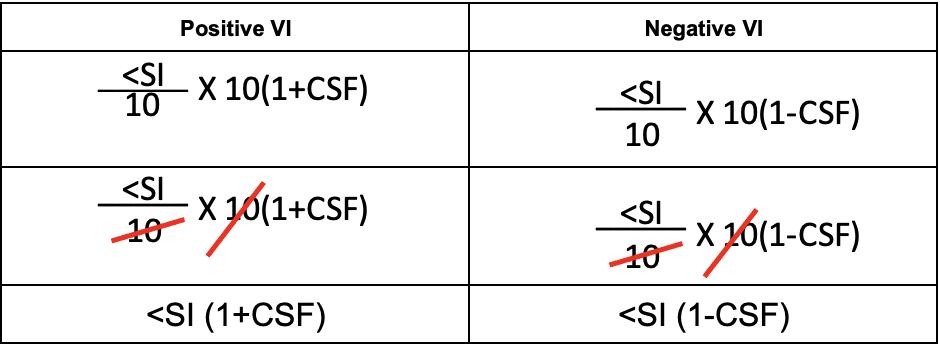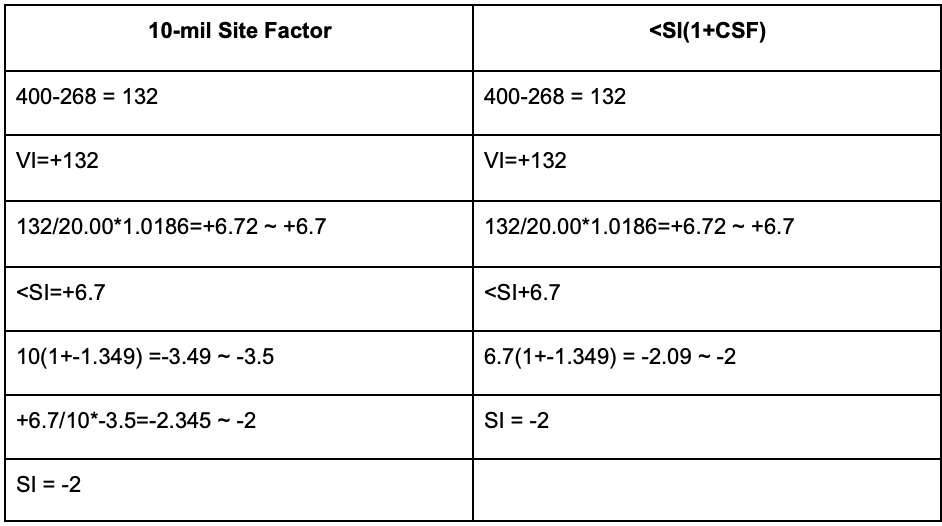# The Field Artillery has a site problem.

The Field Artillery has a site problem.

By: David B. Nance

High Angle Site, the bane of every Basic Officer Leadership Course Lieutenant’s existence. Why is this concept so difficult, and is this small correction even worth calculating? The Field Artillery branch has mulled this calculation for years. To the point where the TC 3-09.81 April 2016 (TC) and the FM 6-40 April 1996 (FM) says that the FDO can ignore high angle site. (Chapter 8-21, 8-12, respectively). I will demonstrate that both high and low angle site are currently unnecessarily complicated and can be solved by one calculation. The site definition by the TC is “the algebraic sum of the angle of site and the complimentary angle of site and is expressed to the nearest mil.” Unneeded complications have crept into the determination of site.

The 1996 FM 6-40 Chapter 8-12 sub para. C states, “High angle site is determined by using the complimentary site factor using the TFT or the 10-mil site factor from the GFT. Using the GFT is the preferred method. The reading from the 10-mil site factor scale is the actual site for each 10 mils of angle of site. The site is computed by multiplying the angle of site divided by 10, by the 10-mil site factor. The 10-mil site factor is always negative.”  The 10-mil site factor gives us the ability to visually extract small values from the GFT that were too small to capture using manual methods before the invention of the modern four-function calculator.

The 2016 TC 3-09.81 states instead that the 10-mil site factor is the preferred method (Chapter 8-23). While the FM 6-40 described the GFT as the preferred method due to its ability to make calculations faster, the TC does not give us the same leeway. The statement that the 10-mil site factor is the preferred method has forced the Artillery into teaching an unnecessarily complicated math formula for manual computations of high angle site. The 10-mil site factor is a useful tool when utilizing the GFT but is an unnecessary step when calculating site manually.

The formula for determining high angle site manually as described by the TC 3-09.81 is:

High Angle Site= (<SI/10) *10 MSF

Breaking this down into individual pieces for a positive vertical interval, we have the following variables:

Vertical Interval (VI) = Target Altitude-Battery Altitude

Angle of Site (<SI) = (VI/RG in 1,000s) * 1.0186

Complimentary Site Factor (CSF): Extracted from the TFT, accounts for non-rigidity in trajectory due to charge and ballistic coefficient.

10 Mil Site Factor (10 MSF) = 10(1+CSF)

Putting this first portion into practice:

Let’s say we have a target altitude of 463, Battery altitude of 438, and a range of 4830 firing a charge 1L projectile HE M795.

463 - 438 = VI = +25.

We then take this VI and divide it by our range in thousands so:

(VI/Range in thousands) *1.0186 = <SI
(+25/4.83) *1.0186 = +5.3

Our angle of site is +5.3, but now we introduce the 10-mil site factor.

The formula for the 10-mil site factor with positive VI is: 10(1+CSF)

We then use Table G from the AR-2 (2016) and extract our CSF.

CSF = -1.176

So then 10(1 + -1.176) = -1.76 ~ 10 Mil Site Factor = -1.8

Finishing it up, we calculate:

(+5.3/10) * -1.8= -0.954 ~ -1

If we build the entire formula using the values, we found we end up with:

(((+25/4.83)/10)1.0186) *10(1+-1.176) = -0.954 ~ -1

If you look at the formula closely you can see that we divide by 10 only to immediately multiply the value by 10 again. Manual computation of the 10-mil site factor adds an unnecessary step and introduces complexity to the math problem.

We can remove that step and use the formula for high angle site=<SI(1+CSF)

Using these same values, we find that:

(+25/4.83)1.0186) (1+-1.176) = -0.928 ~ -1

We end up with the same value for high angle site with a more streamlined math step for both positive and negative vertical interval demonstrated by the following proof:Using the above formulas, we can achieve a more accurate high angle site value due to the reduction of Artillery expression error, making high angle site both faster and more accessible. Notice that the raw data is different prior to the final artillery expression from the whole site value. This is because there is an included Artillery expression error inherent with using the 10-mil site factor. However, using the formula <SI(1+CSF), only two instances of Artillery expressions is needed, increasing accuracy while also increasing the speed of the computation.

Let’s take another look using a larger charge:

Range 20,000, charge 5H M232A1, M795 Shell HE, Target Altitude 400 Battery Altitude 268, High AngleThe Field Artillery must update the TC 3-09.81 Chapter 8 “Site” to show that the 10-mil site factor from the Graphical Firing Table is the preferred method with a positive VI due to the ability to quickly extract values from the GFT. If the target range exceeds the last listed 10-mil site factor, then we will use the last listed value on the GFT. If the target range is below the first listed 10-mil site factor, then we will use <SI(1+CSF). According to the TC, high angle site with a negative VI must is computed manually using <SI(1-CSF).

Low angle site is far less controversial; however, it is also more complicated than it needs to be. The TC states that both high angle site and low angle site are the algebraic sum of the angle of site and the complimentary angle of site and is expressed to the nearest mil. So, if the formula <SI(1+CSF) is true for high angle site it must be universally true for low angle site.

The current low angle site formula is: <SI + complimentary angle of site (CAS).

Positive VI will have a positive <SI and a negative VI will have a negative <SI shown below.

 Positive VI Negative VI

Using the algebraic rule of X+(X*Y) =X(1+Y) we once again find that <SI(1+CSF) appears. Putting this into practice we have:

Range 4500, charge 1L M231, M795 Shell HE, Target Altitude 400 Battery Altitude 268, Low Angle



Range 21,000 Charge 5H 232A1, M795 Shell HE, Target Altitude 200 Battery Altitude 500, Low Angle



As you can see from the above examples, this formula is more efficient and works every single time no matter the inputs. This streamlines both high angle and low angle site into the same easy-to-digest formula. This eliminated the need to have separate formulas for low and high angle site. Not only is this a faster way to compute site, but it is also more accurate. Every time we Artillery Express, inaccuracies are introduced due to “rounding errors.” These improved formulas reduce the number of rounding errors by 33.3% due to the decrease in instances of Artillery Expression. Additionally, this will turn an 8-hour quadrant elevation theory class into a 2-hour block of instruction with an increase in student retention and accuracy.

We must change our site doctrine in all institutions and immediately implement site:

Site Positive VI =<SI(1+CSF)

Site Negative VI <SI(1-CSF)